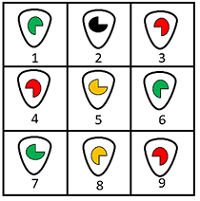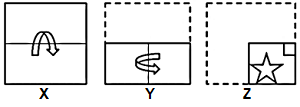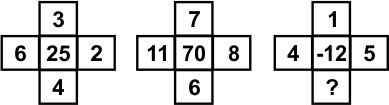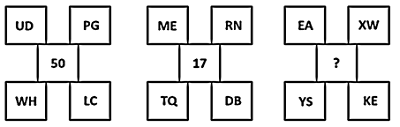# CREST International IQ Challenge Class 3 Sample Papers

## Syllabus:

The IQ Challenge will evaluate students on the following skills:

• Analytical reasoning
• Calculation skills
• Creative thinking
• Information processing
• Lateral thinking
• Observation skills

The questions will be a mix of Basic, Intermediate and Advanced Level. It is advisable that students should attempt questions only if they know the answer. For every incorrect answer, there's a penalty of 1/3rd of the total marks allotted to that question.

 Q.1 Group the given figures into three classes using each figure only once.Q.2 Directions: In the following question, some statements are given followed by some conclusions. Taking the given statements to be true even if they seem to be at variance from commonly known facts, read all the conclusions and then decide which of the given conclusion logically follows the given statements.Statements:I. Some rats are turtles.II. Some turtles are snakes.Conclusions:I. All snakes are rats.II. Some snakes are not turtles.
 Q.3 In a code language, if “ROMANCE” is written as “30”, then how will “GARDEN” be written in that language?
 Q.4 If FLAT is coded 9645, how will DUMB be coded in the same language?
 Q.5 Select the number that can replace the question mark ‘?’ in the following series:24, 30, 36, 42, 52, 60, ?
 Q.6 The sequence of folding a piece of square paper and the manner in which the folded paper has been cut is shown in the figures X, Y and Z. How would this paper look when unfolded?Q.7 Find the missing number.Q.8 Find the missing number.Q.9 Direction: Study the following information carefully and answer the given question The following is the illustration of input and its rearrangement. (Numbers whenever they appear are two-digit numbers) Input: Eat 20 Task 27 Walk 40 21 BagStep I: 20 Eat Task 27 Walk 40 21 BagStep II: 20 21 Eat Task 27 Walk 40 BagStep III: 20 21 27 Eat Task Walk 40 BagStep IV: 20 21 27 40 Eat Task Walk BagStep V: 20 21 27 40 Bag Eat Task WalkAnd step V is the last step of the rearrangement of the above input. As per the rules followed in the above steps, which word or number is 2nd to the left of Kite in Step III?Input: 17 Gun 60 Kite 82 Ink 28 Cat
 Q.10 In each of the following questions, a number series is given. After the series, a number is given followed by(a), (b), (c), (d) and (e). You have to complete the series starting with the number given, following the same logic as that of the original series and answer the questions based on the new series.200, 209, 234, 283, 364, 4859, (a), (b), (c), (d), (e)What is the value of (d)?

Sample PDF of CREST International IQ Challenge for Class 3: